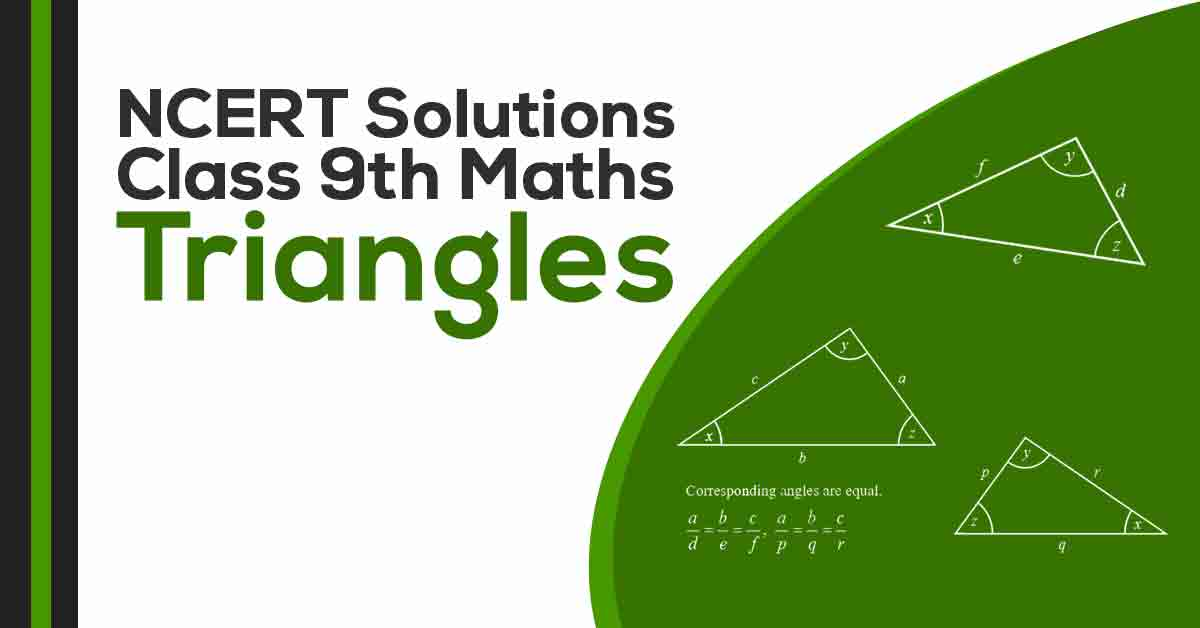NCERT Solutions for Class 9 Maths Chapter 7 | Vidyakul
×

# NCERT Solutions For Class 9 Maths Chapter 7

## NCERT Solutions For Class 9 Maths Chapter 7 Triangles - PDF DownloadStudents require NCERT Solutions for Class 9 Maths Chapter 7 Triangles for better exam preparation.In this chapter, we will study in details about the congruence of triangles, rules of congruence, some more properties of triangles and inequalities in a triangle. Two figures are congruent if they are of the same shape and of the same size. Two circles of the same radii are congruent. Two squares of the same sides are congruent.

These CBSE NCERT Solutions for Class 9 Maths Chapter 7 Triangles will help you to practice all the topics in the chapter with ease and score maximum marks in the examination.